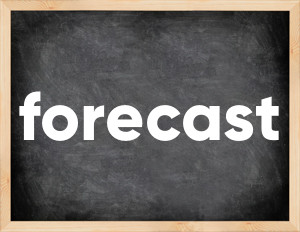# Forecast past tenseThe English verb 'forecast' is pronounced as [ˈfɔːkɑːst].
Related to: irregular verbs, regular verbs.
3 forms of verb forecast: Infinitive (forecast), Past Simple - (forecast), Past Participle - (forecast).

## Here are the past tense forms of the verb forecast

👉 Forms of verb forecast in future and past simple and past participle.
❓ What is the past tense of forecast.

## Forecast: Past, Present, and Participle Forms

Base Form Past Simple Past Participle
forecast [ˈfɔːkɑːst]

forecast [ˈfɔːkɑːst]

forecasted [ˈfɔːkɑːstɪd]

forecast [ˈfɔːkɑːst]

forecasted [ˈfɔːkɑːstɪd]

## What are the 2nd and 3rd forms of the verb forecast?

🎓 What are the past simple, future simple, present perfect, past perfect, and future perfect forms of the base form (infinitive) 'forecast'?

### Learn the three forms of the English verb 'forecast'

• the first form (V1) is 'forecast' used in present simple and future simple tenses.
• the second form (V2) is 'forecast', 'forecasted' used in past simple tense.
• the third form (V3) is 'forecast', 'forecasted' used in present perfect and past perfect tenses.

## What are the past tense and past participle of forecast?

The past tense and past participle of forecast are: forecast in past simple is forecast, forecasted, and past participle is forecast. forecasted.

### What is the past tense of forecast?

The past tense of the verb "forecast" is "forecast", or "forecasted", and the past participle is "forecast" or "forecasted".

### Verb Tenses

Past simple — forecast in past simple forecast, forecasted (V2).
Future simple — forecast in future simple is forecast (will + V1).
Present Perfect — forecast in present perfect tense is forecast, forecasted (have/has + V3).
Past Perfect — forecast in past perfect tense is forecast, forecasted (had + V3).

### forecast regular or irregular verb?

👉 Is 'forecast' a regular or irregular verb? The verb 'forecast' is regular or irregular verb.

## Examples of Verb forecast in Sentences

•   The government is forecasting reduced budget revenues (Present Continuous)
•   Paul forecast that an earthquake would occur soon (Past Simple)
•   They forecast rain for next week (Past Simple)
•   Who is able to forecast currency exposures? (Present Simple)
•   They are forecasting that retail sales will rise further in 2021 (Present Continuous)
•   He's forcasted the crisis, what should we do? (Present Perfect)
•   It is still difficult for scientists to forecast the rate of species change (Present Simple)
•   Will he forcast what will be the trade topic next year? (Future Simple)
•   Nobody will forecast the exchange rate for next six months (Future Simple)
•   They accurately forecasted social spendings (Past Simple)

Along with forecast, words are popular chase and hate.

Verbs by letter: , , , , , , , , , , , , , , , , , , , , , , , , .